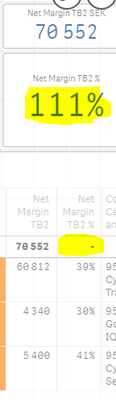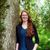Announcements
Applications are open for the 2024 Qlik Luminary Program. Apply by December 15 here.
cancel
Showing results for
Did you mean:Contributor III

## Formula for Net Margin problems

This is my Net Margin Formula:

Net Margin = sum(aggr(((([Hourly Sales Rate]*[No of Charged Hours])-(([Wage Cost per Hour]+[Overhead Cost per Hour])*[No of Charged Hours]))/([Hourly Sales Rate]*[No of Charged Hours])),[Income Travel],[Hourly Sales Rate], Period,[Project Activity Nr and Name],[Project Nr and Name],[Cost Centre and Name]))

It works perfectly. The problem is that I need a Net Margin %

Net Margin % =Net Margin/([Hourly Sales Rate]*[No of Charged Hours])

When I add this I get the correct %-age on the rows, but not as totals???Why is this and what can I do?

1 Solution

Accepted SolutionsMVP

You have no aggregation function around your denominator--if you add a sum() around it, the value will appear.  You will need to determine if the result is what you are expecting or not though.

2 RepliesMVP

You have no aggregation function around your denominator--if you add a sum() around it, the value will appear.  You will need to determine if the result is what you are expecting or not though.Contributor III
Author

Thank you thank you thank you! YEEESSS! now its perfect 💃🙏Tags
Community Browser